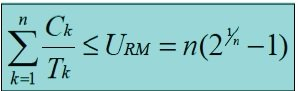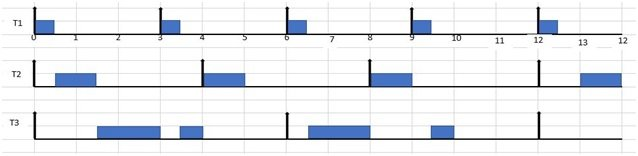# RATE MONOTONIC (RM) SCHEDULING ALGORITHM

The Rate Monotonic scheduling algorithm is a simple rule that assigns priorities to different tasks according to their time period. That is task with smallest time period will have highest priority and a task with longest time period will have lowest priority for execution. As the time period of a task does not change so not its priority changes over time, therefore Rate monotonic is fixed priority algorithm. The priorities are decided before the start of execution and they does not change overtime.

### introduction to RATE MONOTONIC (RM) SCHEDULING ALGORITHM

Rate monotonic scheduling Algorithm works on the principle of preemption. Preemption occurs on a given processor when higher priority task blocked lower priority task from execution. This blocking occurs due to priority level of different tasks in a given task set.  rate monotonic is a preemptive algorithm which means if a task with shorter period comes during execution it will gain a higher priority and can block or preemptive currently running tasks. In RM priorities are assigned according to time period. Priority of a task is inversely proportional to its timer period. Task with lowest time period has highest priority and the task with highest period will have lowest priority.  A given task is scheduled under rate monotonic scheduling Algorithm, if its satisfies the following equation:### Example of RATE MONOTONIC (RM) SCHEDULING ALGORITHM

For example, we have a task set that consists of three tasks as follows

 Tasks Release time(ri) Execution time(Ci) Deadline (Di) Time period(Ti) T1 0 0.5 3 3 T2 0 1 4 4 T3 0 2 6 6

U= 0.5/3 +1/4 +2/6 = 0.167+ 0.25 + 0.333 = 0.75

As processor utilization is less than 1 or 100% so task set is schedulable and it also satisfies the above equation of rate monotonic scheduling algorithm.Figure 1. RM scheduling of Task set in table 1.

A task set given in table 1 it RM scheduling is given in figure 1. The explanation of above is as follows

1. According to RM scheduling algorithm task with shorter period has higher priority so T1 has high priority, T2 has intermediate priority and T3 has lowest priority. At t=0 all the tasks are released. Now T1 has highest priority so it executes first till t=0.5.
2. At t=0.5 task T2 has higher priority than T3 so it executes first for one-time units till t=1.5. After its completion only one task is remained in the system that is T3, so it starts its execution and executes till t=3.
3. At t=3 T1 releases, as it has higher priority than T3 so it preempts or blocks T3 and starts it execution till t=3.5. After that the remaining part of T3 executes.
4. At t=4 T2 releases and completes it execution as there is no task running in the system at this time.
5. At t=6 both T1 and T3 are released at the same time but T1 has higher priority due to shorter period so it preempts T3 and executes till t=6.5, after that T3 starts running and executes till t=8.
6. At t=8 T2 with higher priority than T3 releases so it preempts T3 and starts its execution.
7. At t=9 T1 is released again and it preempts T3 and executes first and at t=9.5 T3 executes its remaining part. Similarly, the execution goes on.